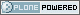# Neuroimaging Informatics Technology Initiative

##### Personal tools
You are here: Home » dim_info.html

# dim_info.html

## dim_info

• Links to FAQ questions
• Links to Bboard questions

### nifti1.h header documentation

```/---------------------------------------------------------------------------/
/* MRI-SPECIFIC SPATIAL AND TEMPORAL INFORMATION:
---------------------------------------------
A few fields are provided to store some extra information
that is sometimes important when storing the image data
from an FMRI time series experiment.  (After processing such
data into statistical images, these fields are not likely
to be useful.)  { freq_dim  } = These fields encode which spatial dimension (1,2, or 3)
{ phase_dim } = corresponds to which acquisition dimension for MRI data.
{ slice_dim } =
Examples:
Rectangular scan multi-slice EPI:
freq_dim = 1  phase_dim = 2  slice_dim = 3  (or some permutation)
Spiral scan multi-slice EPI:
freq_dim = phase_dim = 0  slice_dim = 3
since the concepts of frequency- and phase-encoding directions
don't apply to spiral scan    slice_duration = If this is positive, AND if slice_dim is nonzero,
indicates the amount of time used to acquire 1 slice.
slice_duration*dim[slice_dim] can be less than pixdim
with a clustered acquisition method, for example.
slice_code = If this is nonzero, AND if slice_dim is nonzero, AND
if slice_duration is positive, indicates the timing
pattern of the slice acquisition.  The following codes
are defined:
NIFTI_SLICE_SEQ_INC
NIFTI_SLICE_SEQ_DEC
NIFTI_SLICE_ALT_INC
NIFTI_SLICE_ALT_DEC
{ slice_start } = Indicates the start and end of the slice acquisition
{ slice_end   } = pattern, when slice_code is nonzero.  These values
are present to allow for the possible addition of
"padded" slices at either end of the volume, which
don't fit into the slice timing pattern.  If there
are no padding slices, then slice_start=0 and
slice_end=dim[slice_dim]-1 are the correct values.
For these values to be meaningful, slice_start must
be non-negative and slice_end must be greater than
slice_start.

The following table indicates the slice timing pattern, relative to
time=0 for the first slice acquired, for some sample cases.  Here,
dim[slice_dim]=7 (there are 7 slices, labeled 0..6), slice_duration=0.1,
and slice_start=1, slice_end=5 (1 padded slice on each end).    slice
index   SEQ_INC SEQ_DEC ALT_INC ALT_DEC
6  --   n/a     n/a     n/a     n/a     n/a = not applicable
5  --   0.4     0.0     0.2     0.0           (slice time offset
4  --   0.3     0.1     0.4     0.3            doesn't apply to
3  --   0.2     0.2     0.1     0.1            slices outside range
2  --   0.1     0.3     0.3     0.4            slice_start..slice_end)
1  --   0.0     0.4     0.0     0.2
0  --   n/a     n/a     n/a     n/a

The fields freq_dim, phase_dim, slice_dim are all squished into the single
byte field dim_info (2 bits each, since the values for each field are
limited to the range 0..3).  This unpleasantness is due to lack of space
in the 348 byte allowance.
The macros DIM_INFO_TO_FREQ_DIM, DIM_INFO_TO_PHASE_DIM, and
DIM_INFO_TO_SLICE_DIM can be used to extract these values from the
dim_info byte.
The macro FPS_INTO_DIM_INFO can be used to put these 3 values
into the dim_info byte.

```

### DEFINED CODES

```#define NIFTI_SLICE_UNKNOWN  0
#define NIFTI_SLICE_SEQ_INC  1
#define NIFTI_SLICE_SEQ_DEC  2
#define NIFTI_SLICE_ALT_INC  3
#define NIFTI_SLICE_ALT_DEC  4
```

### MISCELLANEOUS C MACROS

```#define DIM_INFO_TO_FREQ_DIM(di)   ( ((di)     ) & 0x03 )
#define DIM_INFO_TO_PHASE_DIM(di)  ( ((di) >> 2) & 0x03 )
#define DIM_INFO_TO_SLICE_DIM(di)  ( ((di) >> 4) & 0x03 )
#define FPS_INTO_DIM_INFO(fd,pd,sd) ( ( ( ((char)(fd)) & 0x03)      ) |  \
( ( ((char)(pd)) & 0x03) << 2 ) |  \
( ( ((char)(sd)) & 0x03) << 4 )  )
```

Created by Alex Clark